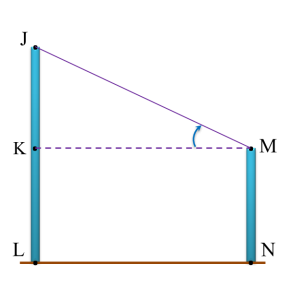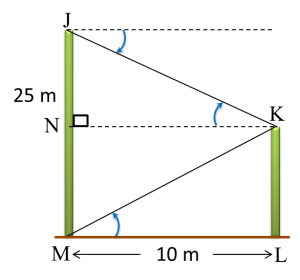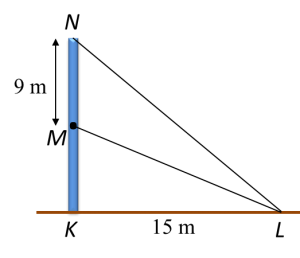# 10.3.1 Angles of Elevation and Depression, Paper 1 (Short Questions)

Question 1:
Diagram below shows two vertical poles on a horizontal plane. J, K, L, M and N are five points on the poles such that KL = MN.Name the angle of elevation of point J from point M.

Solution:Angle of elevation of point J from point M = ∠KMJ

Question 2:
Diagram below shows two vertical poles JM and KL, on a horizontal plane.The angle of depression of vertex K from vertex J is 42o.
Calculate the angle of elevation of vertex K from M.

Solution:JN = 10 tan 42o = 9.004 m
NM = 25 – 9.004 = 15.996 m
KL = NM = 15.996 m
$\begin{array}{l}\mathrm{tan}\angle KML=\frac{KL}{ML}\\ \text{}=\frac{15.996}{10}\\ \text{}=1.5996\\ \angle KML={\mathrm{tan}}^{-1}1.5996\\ \text{}={57}^{o}{59}^{‘}\end{array}$

Question 3:
Diagram below shows a vertical pole KMN on a horizontal plane. The angle of elevation of from L is 20o.Calculate the height, in m, of the pole.

Solution:$\begin{array}{l}\mathrm{tan}{20}^{o}=\frac{KM}{KL}\\ 0.3640=\frac{KM}{15}\\ KM=5.4m\\ \text{Height of the pole =}9+5.4=14.4m\end{array}$